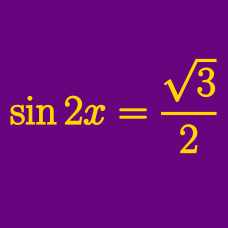Geometry

# Specific Solution of Simple Trigonometric Equations

How many solutions are there to $\sin \theta + 7 = 8$ in the domain $[0 ^ \circ, 1000 ^ \circ ]$?

What is the minimum value of a positive number $x$ such that the value of $\sin(x+\frac{\pi}{8})$ is $0?$

How many $x$'s in the interval $0 \leq x \leq 15\pi$ satisfy $\tan x=-\sqrt{3}$?

What are the solutions to $12\sin \left( 2x-\frac{7}{6}\pi \right)=6\sqrt{2}$ in the interval $x \in [0,\pi]?$

Which of the following is a solution to

$\sin ( 2 \theta - 31 ^ \circ ) = \cos 49 ^ \circ ?$

×